# Dimension Demonstration

Compute the dimension of the attractor of the Hénon map

## Compute the Hénon attractor with the standard values

a=1.4;
b=0.3;
n=10000;
nTransient=100;
x=zeros(2,n+1);
for k=1:n+nTransient
x(:,k+1)=henon(x(:,k),a,b);
end
x=x(:,nTransient+1:end);
y=x(2,:);
x=x(1,:);
plot(x,y,'.','markersize',4)
axis equal tight off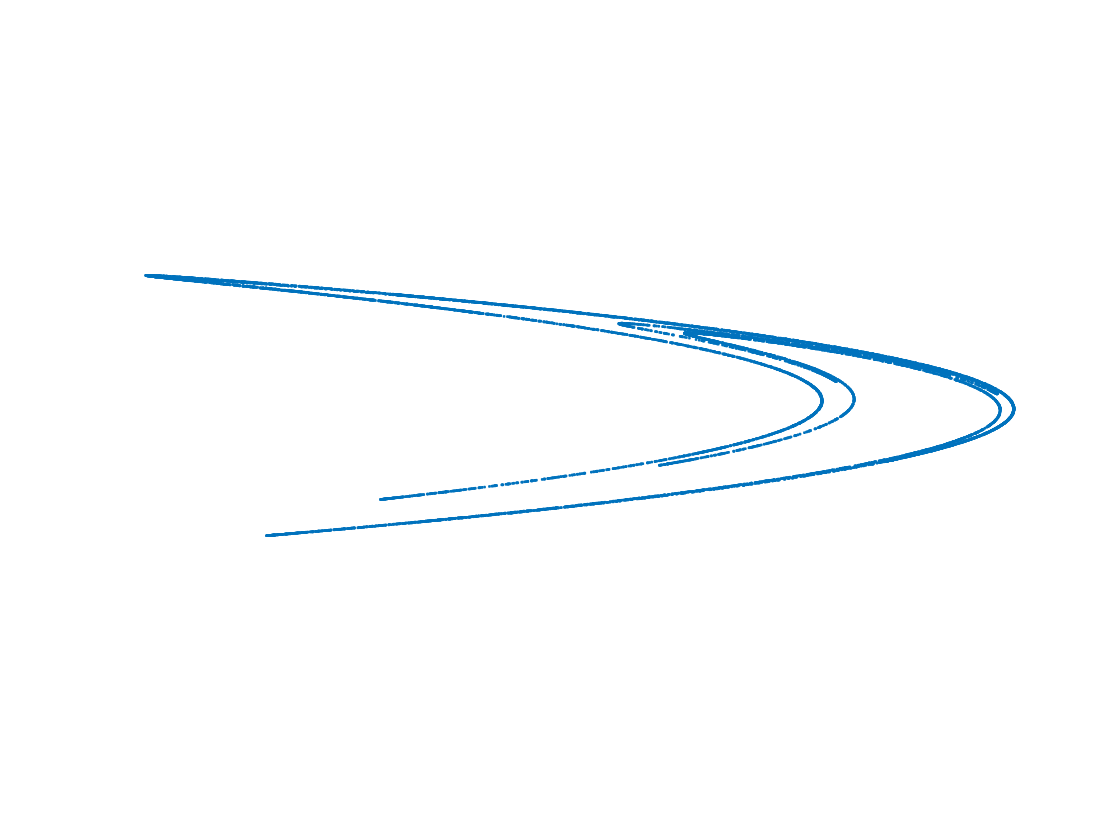## Box-counting step

N=7;
epsilon=zeros(1,N);
Nboxes=zeros(1,N);
for k=1:N
K=2^(k+1);
[epsilon(k),Nboxes(k)]=countAndPlotBoxes(x,y,K);
end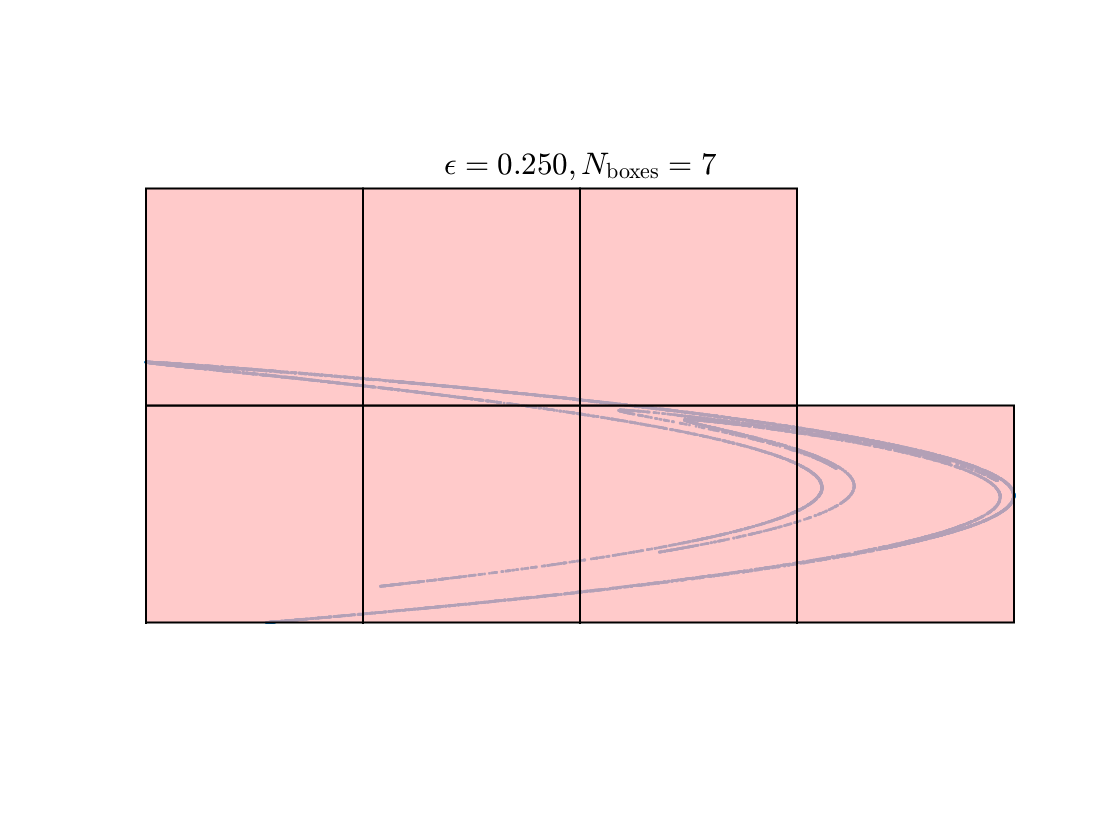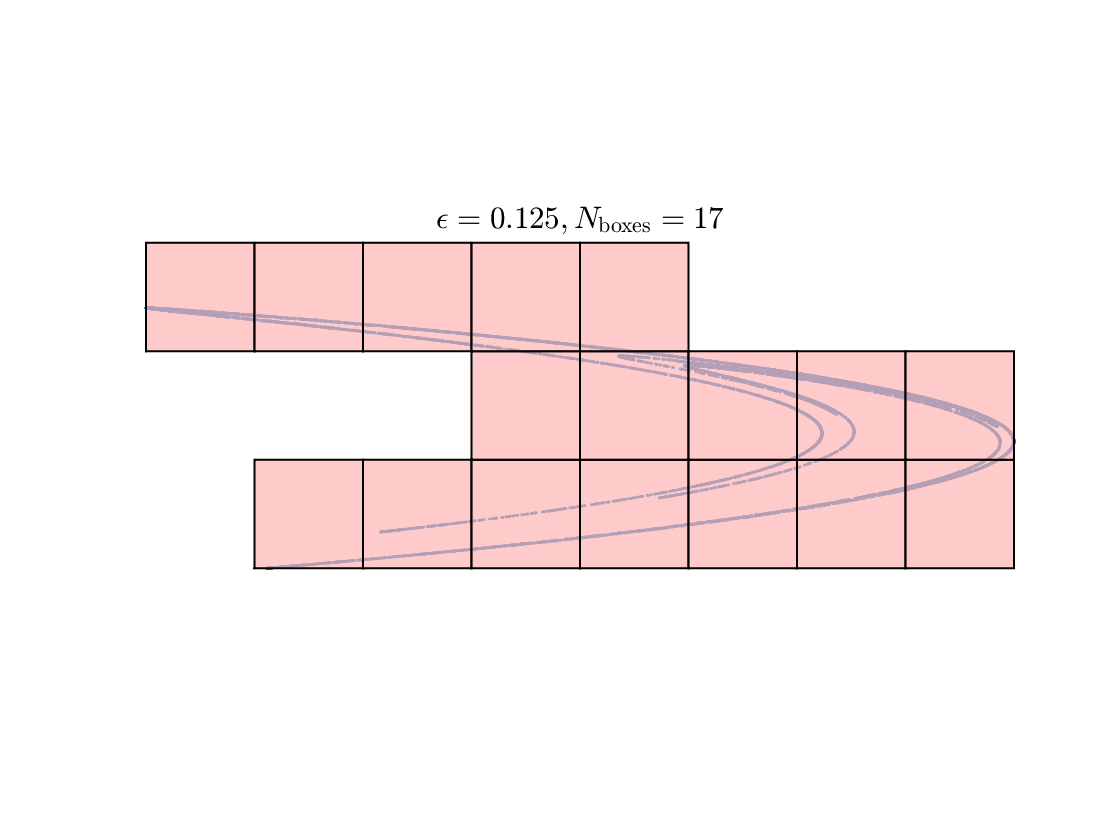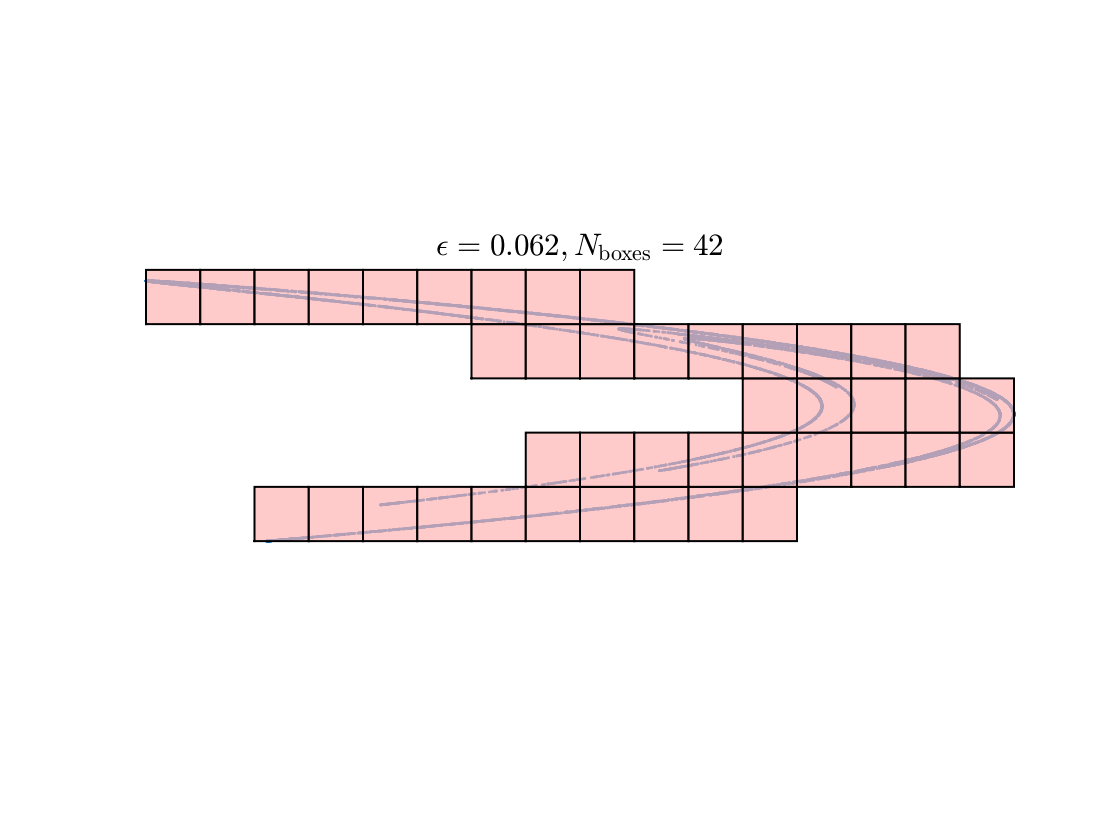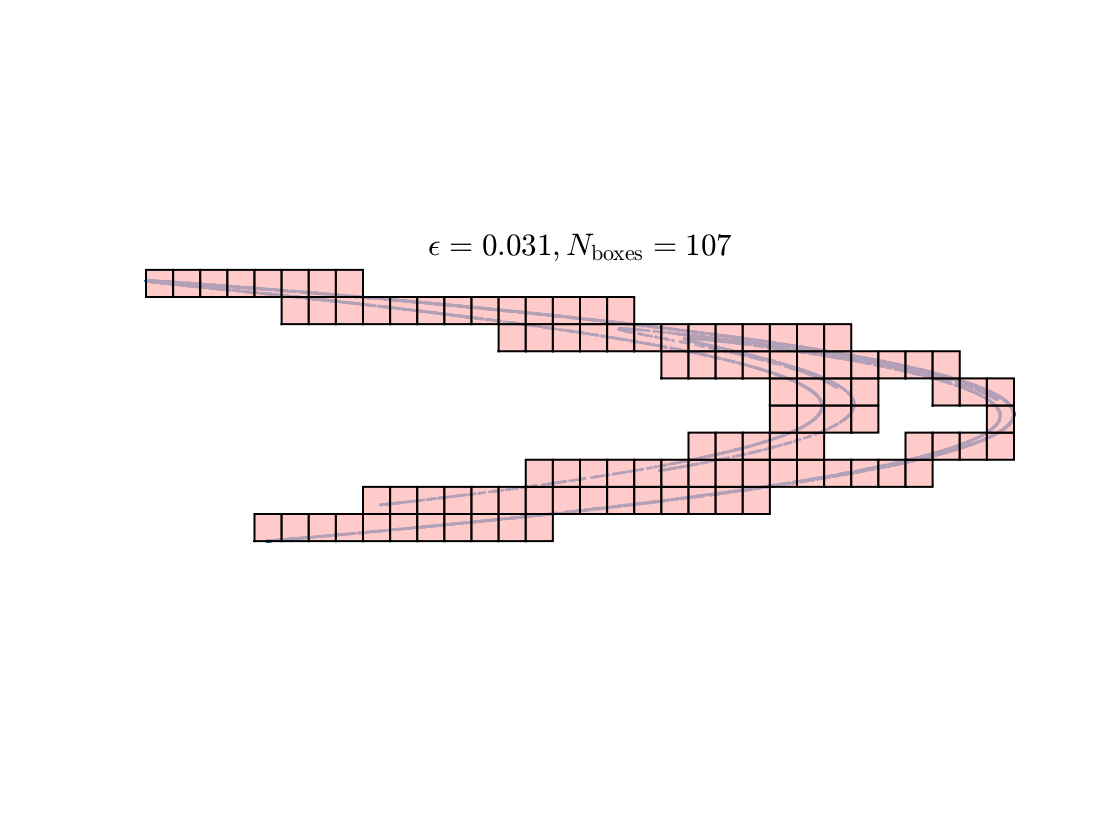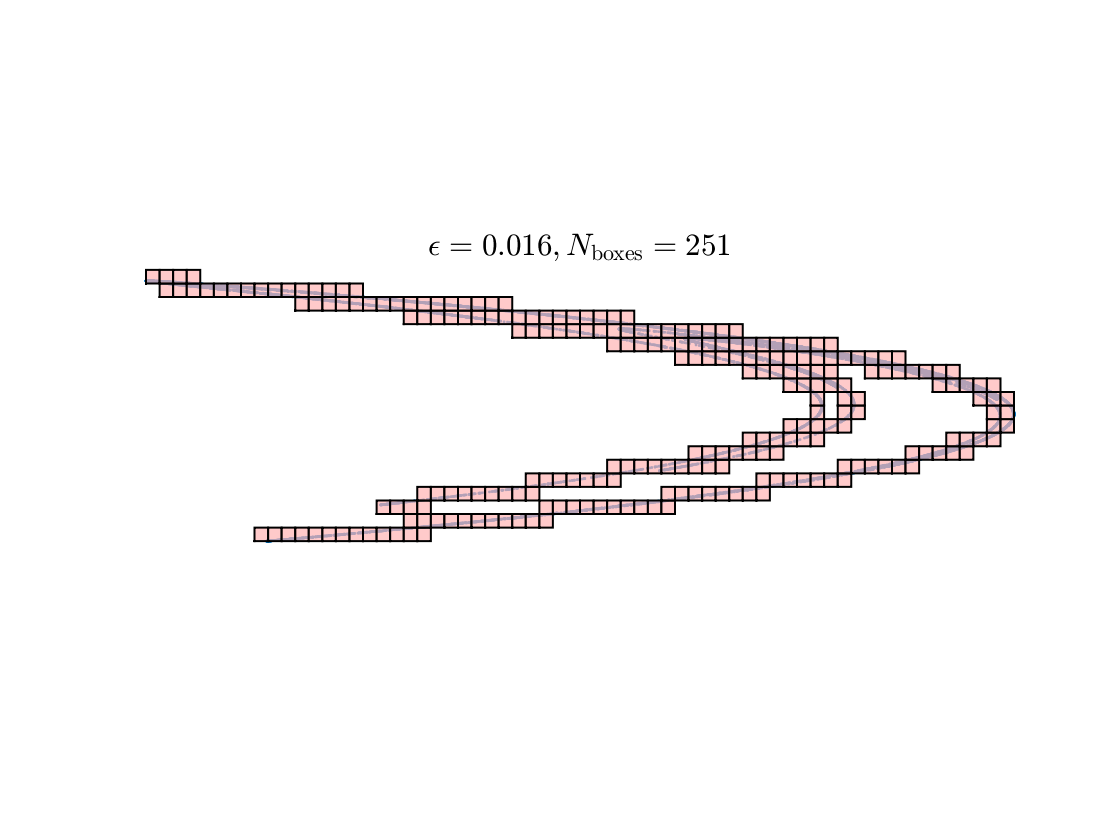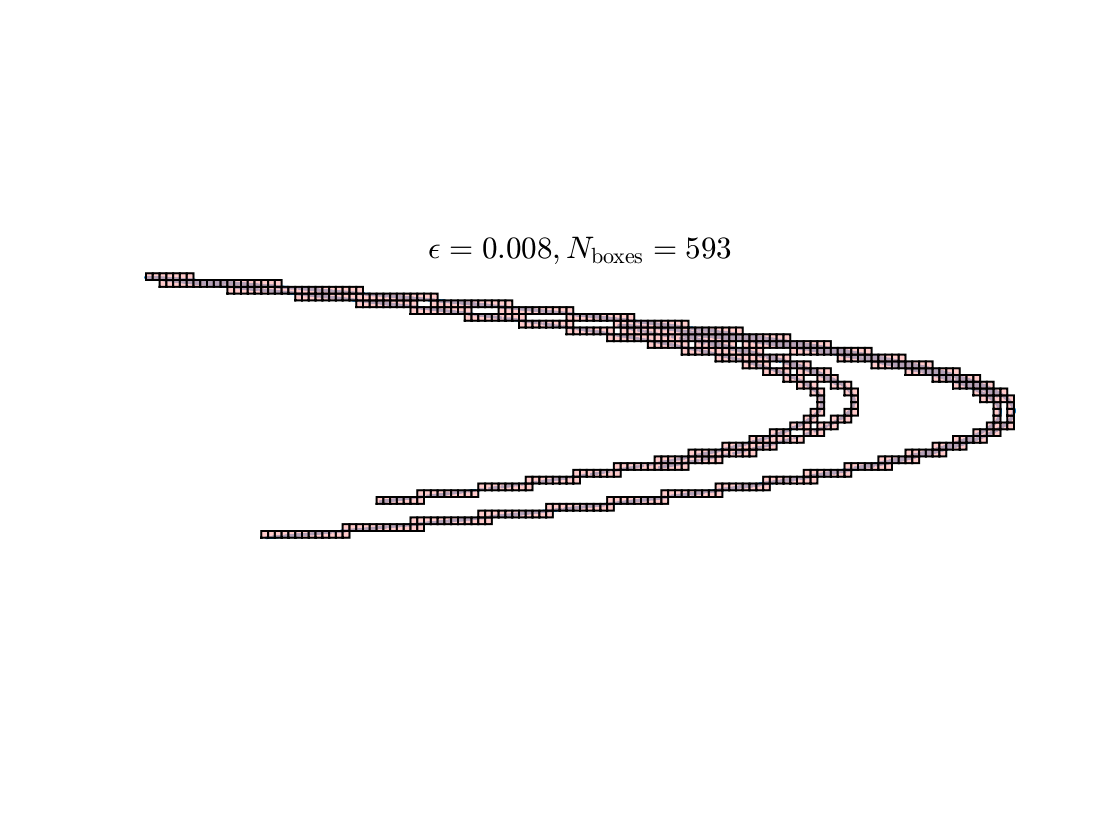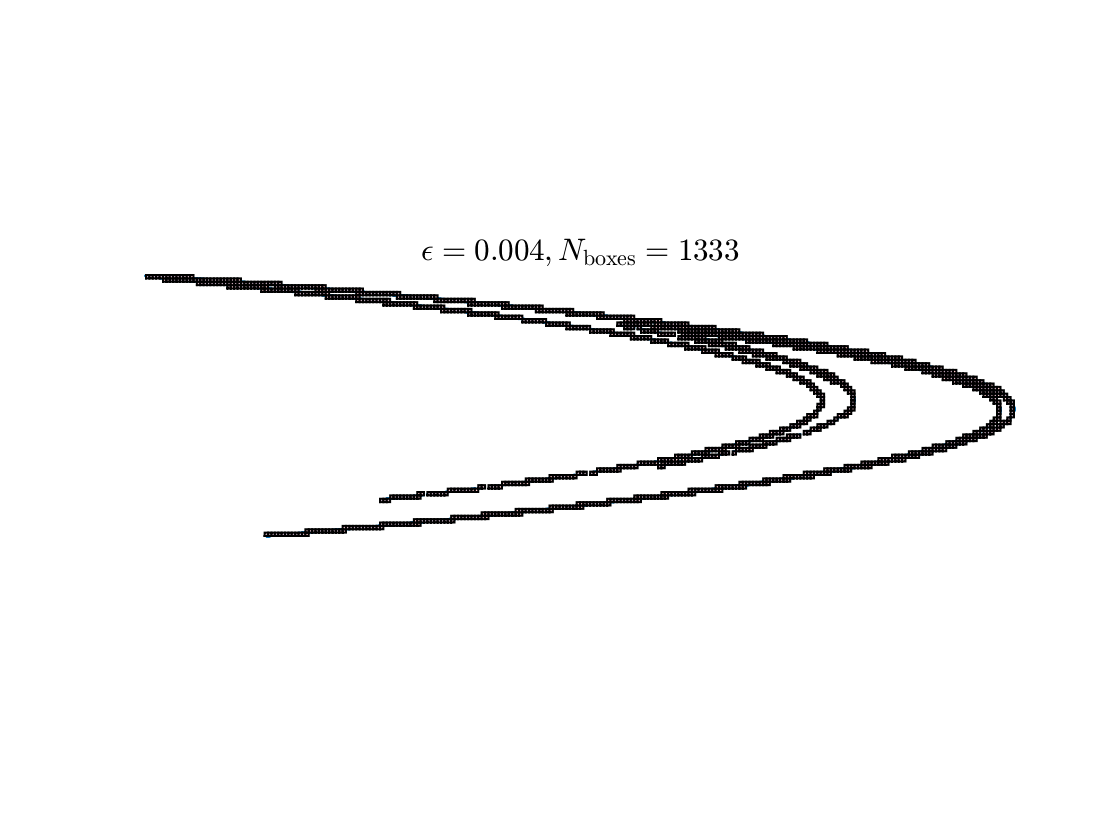## Processing the box-counting step

clf; hold on;
p=polyfit(log(epsilon),log(Nboxes),1);
dim=abs(p(2));
loglog(epsilon,Nboxes,'o',epsilon,exp(polyval(p,log(epsilon))));
set(gca,'xscale','log','yscale','log')
xlabel('$\epsilon$')
ylabel('$N(\epsilon)$')
legend('data','least squares line');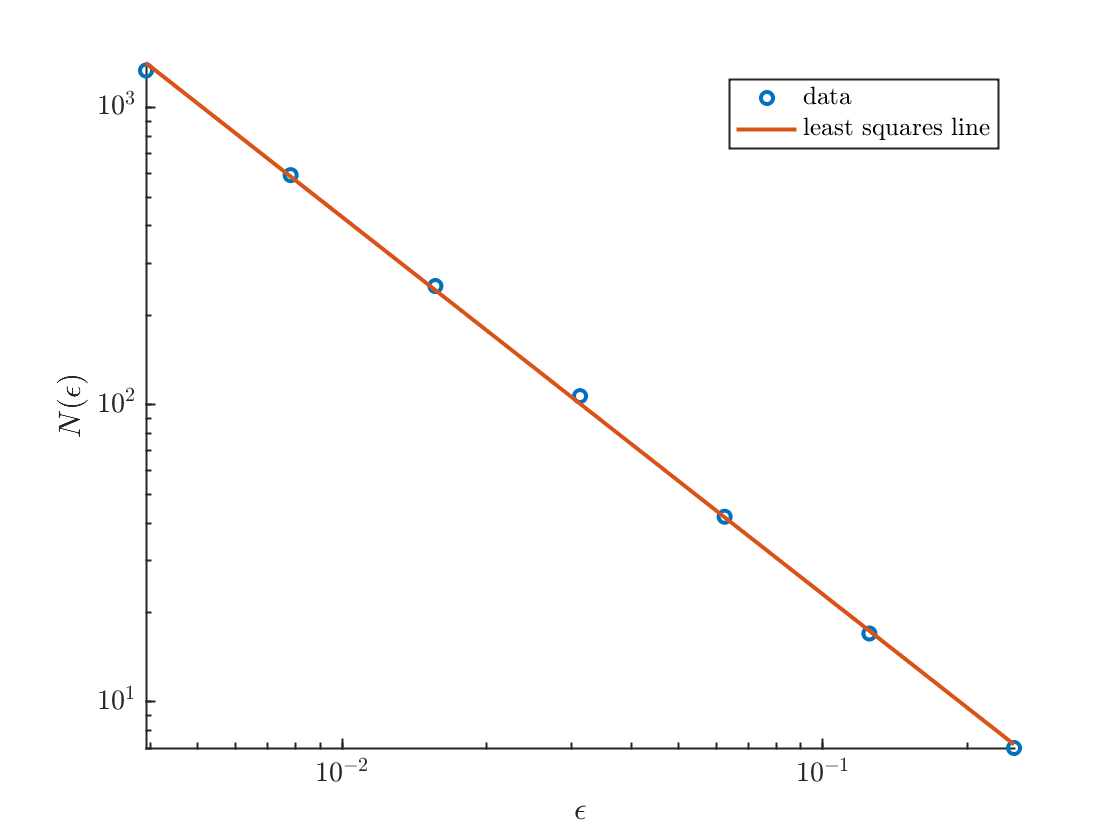fprintf('The calculated dimension is %0.2f.\n',abs(p(1)))
The calculated dimension is 1.27.

## Definition of the Hénon map

The Hénon map, most commonly studied with a=1.4, b=.3
function X=henon(x,a,b)
y=x(2,:);x=x(1,:);
X=[1-a*x.^2+y
b*x];
end

## Function to count boxes for fixed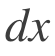function [dx,Nboxes]=countAndPlotBoxes(x,y,K)
Scale and reposition the point set so that it fits in the box [0,1]x[0,1]
xmax=max(x); xmin=min(x); width=xmax-xmin;
ymax=max(y); ymin=min(y); height=ymax-ymin;
L=max(height,width);
y=(y-ymin)/L;
x=(x-xmin)/L;
Define the grid size
dx=1/K;
Define a square box with unit-length sides
xbox=[0 1 1 0 0];
ybox=[0 0 1 1 0];
Plot the point set
figure;clf; hold on;
plot(x,y,'.','markersize',4)

#### The main step:

Loop through all the sub-boxes of length and width dx. Set isFilled(row,col) to 1 if there is a point in the box, and to zero if not. If it is filled, plot a pink square.
Note that this makes use of two built-in MATLAB commands
• inpolygon tests whether each point (x(row,col),y(row,col)) is in the box defined by xv and yv. This is vectorized and avoids the need for loops. (Not something you'd use very often.)
• any returns 1 if any elements of a matrix are nonzero and returns 0 otherwise. The all command is similar. These are very useful utilities.
isFilled=zeros(K,K);
for row=1:K
for col=1:K
xv=(xbox+col-1)*dx;
yv=(ybox+row-1)*dx;
isFilled(row,col)=any(inpolygon(x,y,xv,yv));
if isFilled(row,col)
patch(xv,yv,zeros(1,5),'facecolor',[1 .7 .7],'edgecolor','k','facealpha',0.7)
end
end
end
Nboxes=sum(isFilled(:)); %count the number of filled boxes
axis equal tight off
title(sprintf('$\\epsilon=%0.3f, N_{\\rm boxes}=%i$',dx,Nboxes))
end